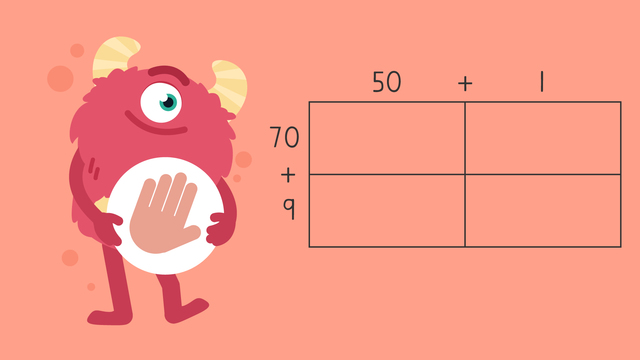# Multiplying Two-Digit Numbers by Two-Digit Numbers Using an Area Model—Let's PracticeRating

Ø 5.0 / 2 ratings
The authorsTeam Digital
Multiplying Two-Digit Numbers by Two-Digit Numbers Using an Area Model—Let's Practice
CCSS.MATH.CONTENT.4.NBT.B.5

## Basics on the topicMultiplying Two-Digit Numbers by Two-Digit Numbers Using an Area Model—Let's Practice

Today we are practicing multiplying 2-digit numbers by 2-digit numbers using an area model with Razzi! This video contains examples to help you further practice and grow confident in this topic.

### TranscriptMultiplying Two-Digit Numbers by Two-Digit Numbers Using an Area Model—Let's Practice

Razzi says get these items ready (...) Because today we're going to practice... Multiplying Two-Digit Numbers by Two-Digit Numbers Using an Area Model. It's time to begin! Use an area model to solve... fifty-one times seventy-nine. Pause the video to work on the problem (...) and press play when you are ready to see the solution! First, set up the area model by labeling the top and left side with each factor written in expanded form. Then multiply, (...) fifty times seventy (...) which equals three thousand five hundred... fifty times nine (...) which equals four hundred fifty... one times seventy (...) which equals seventy... and one times nine (...) which equals nine. Last add the partial products. Did you also get four thousand twenty-nine? Let's tackle the next problem! Use an area model to solve... twenty-three times eighty-six. Pause the video to work on the problem (...) and press play when you are ready to see the solution! First, set up the area model by labeling the top and left side with each factor written in expanded form. Then multiply, (...) twenty times eighty (...) which equals one thousand six hundred... twenty times six (...) which equals one hundred twenty... three times eighty (...) which equals two hundred forty... and three times six (...) which equals eighteen. Last add the partial products. Did you also get one thousand nine hundred seventy-eight? Razzi had so much fun practicing with you today! See you next time!

## Multiplying Two-Digit Numbers by Two-Digit Numbers Using an Area Model—Let's Practice exercise

Would you like to apply the knowledge you’ve learned? You can review and practice it with the tasks for the video Multiplying Two-Digit Numbers by Two-Digit Numbers Using an Area Model—Let's Practice.
• ### Which option is the correct expanded form of 97?

Hints

Remember, expanded form will show each digit expanded into its actual value.

Take a look at this example of the number 23 written in expanded form!

Solution

The correct way to write the number 97 in expanded form is 90 + 7! To do that we separated the number into the tens digit and the ones digit.

• ### Solve 24 x 12.

Hints

Remember the steps to solve the problem!

1) Set up the area model by labeling the top and left side with each factor written in expanded form.

2) Multiply each square in the area model.

Here is the correct area model for this problem to help.

Solution

The correct answer is 288! In this image, we see all of the multiplication problems done correctly. Then once we add up all the answers (200 + 40 + 40 + 8), we get the correct answer.

• ### Solve 86 x 54.

Hints

Remember the steps to solve the problem!

1) Set up the area model by labeling the top and left side with each factor written in expanded form.

2) Multiply each square in the area model.

Here is the correct area model for this problem to help.

Solution

The correct answer is 4,644! In this image, we see all of the multiplication problems done correctly. Then once we add up all the answers (4,000 + 300 + 320 + 24), we get the correct answer!

• ### 79 x 67

Hints

Remember the steps to solve the problem!

1) Set up the area model by labeling the top and left side with each factor written in expanded form.

2) Multiply each square in the area model.

Here is the correct area model for this problem to help!

Solution

The correct answer is 5,293! In this photo, we see all of the multiplication problems done correctly. Then once we add up all the answers (4200 + 540 + 490 + 63), we get the correct answer!

• ### Solve 45 x 21.

Hints

Remember the steps to solve the problem!

1) Set up the area model by labeling the top and left side with each factor written in expanded form.

2) Multiply each square in the area model.

Your area model should look like in the picture!

Solution

The correct answer is 945! In this image, we see all of the multiplication problems done correctly. Then once we add up all the answers (800 + 100 + 40 + 5), we get the correct answer!

• ### 98 x 86

Hints

Remember the steps to solve the problem!

1) Set up the area model by labeling the top and left side with each factor written in expanded form.

2) Multiply each square in the area model.